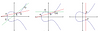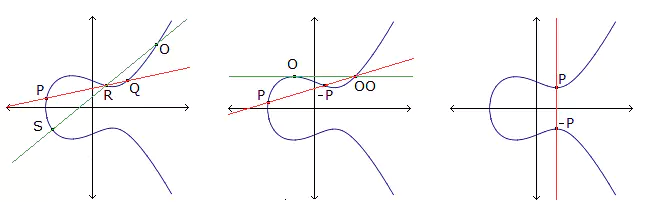# Arcs in Desarguesian nets

### Journal article

A. Beato, G. Faina, M. Giulietti
Contributions Discret. Math., 2008

Semantic Scholar DBLP DOI
Cite

### Cite

APA
Beato, A., Faina, G., & Giulietti, M. (2008). Arcs in Desarguesian nets. Contributions Discret. Math.

Chicago/Turabian
Beato, A., G. Faina, and M. Giulietti. “Arcs in Desarguesian Nets.” Contributions Discret. Math. (2008).

MLA
Beato, A., et al. “Arcs in Desarguesian Nets.” Contributions Discret. Math., 2008.

## Abstract

A trivial upper bound on the size k of an arc in an r-net is $k \leq r + 1$. It has been known for about 20 years that if the r-net is Desarguesian and has odd order, then the case $k = r + 1$ cannot occur, and $k \geq r - 1$ implies that the arc is contained in a conic. In this paper, we show that actually the same must hold provided that the difference $r - k$ does not exceed $\sqrt{k/18}$. Moreover, it is proved that the same assumption ensures that the arc can be extended to an oval of the net.

Share